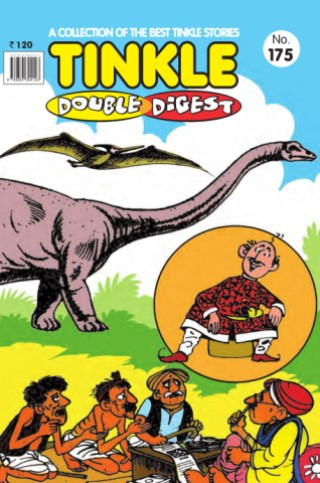## Tinkle Double Digest No 175(2)
• Description
• Author
• Info
• Reviews

### Description

Tinkle Double Digest No. 175

### Reviews

Aditya Kiran Joseph rated on Tinkle Double Digest No. 175 from Tinkle Double Digest No. 175

kp likes Tinkle Double Digest No. 175 from Tinkle Double Digest No. 175
kp loves Tinkle Double Digest No. 175 from Tinkle Double Digest No. 175
kp commented on Tinkle Double Digest No. 175 from Tinkle Double Digest No. 175: awesome = = = = = = =;=;=�=�=�=�=�=�=�=�=�=�=�=�=�=�=�=�=�=�=�=�=�=�=�=�=�=�=�=�=�=�=�=�=�=�=�=�=�=�=�=�=�=�=�=�=�=�=k=k=k=k=k=k=k=k=k=k=k=k=k=�=�=�ddd=�=�=�=�=�=�=�=�=�=�=�=�=�=�=�=�=�=�=�=�=�=�=�=�=�=�=�=�=�=�=�=�=�=�=�=�=�=�=�=�=�
Johaan Philip likes Tinkle Double Digest No. 175 from Tinkle Double Digest No. 175